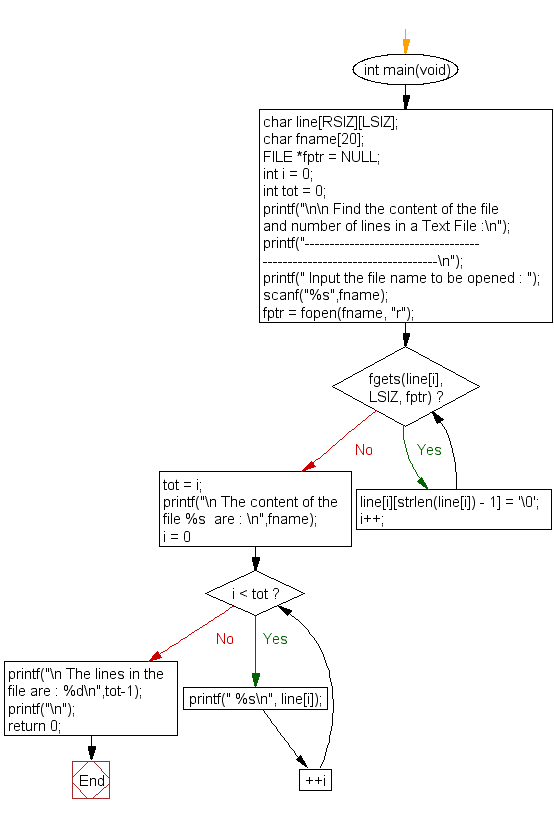﻿ C : Get the number of lines and content of a text file# C Exercises: Find the content of the file and number of lines in a Text File

## C File Handling : Exercise-6 with Solution

Write a program in C to find the content of the file and number of lines in a Text File.

Sample Solution:

C Code:

``````#include <stdio.h>
#include <stdlib.h>
#include <string.h>

#define LSIZ 128
#define RSIZ 10

int main(void)
{
char line[RSIZ][LSIZ];
char fname;
FILE *fptr = NULL;
int i = 0;
int tot = 0;
printf("\n\n Find the content of the file and number of lines in a Text File :\n");
printf("----------------------------------------------------------------------\n");
printf(" Input the file name to be opened : ");
scanf("%s",fname);

fptr = fopen(fname, "r");
/*--------------------- store the lines into an array ----------------*/
while(fgets(line[i], LSIZ, fptr))
{
line[i][strlen(line[i]) - 1] = '\0';
i++;
}
tot = i;
printf("\n The content of the file %s  are : \n",fname);
for(i = 0; i < tot; ++i)
{
printf(" %s\n", line[i]);
}
/*---------------------------------------------------------------------*/
printf("\n The lines in the file are : %d\n",tot-1);
printf("\n");

return 0;
}
```
```

Sample Output:

``` Find the content of the file and number of lines in a Text File :
----------------------------------------------------------------------
Input the file name to be opened : test.txt

The content of the file test.txt  are :

test line 1
test line 2
test line 3
test line 4

The lines in the file are : 4
```

Flowchart:C Programming Code Editor:

Have another way to solve this solution? Contribute your code (and comments) through Disqus.

What is the difficulty level of this exercise?

Test your Programming skills with w3resource's quiz.

﻿

## C Programming: Tips of the Day

What is the size of an enum in c?

An enum is only guaranteed to be large enough to hold int values. The compiler is free to choose the actual type used based on the enumeration constants defined so it can choose a smaller type if it can represent the values you define. If you need enumeration constants that don't fit into an int you will need to use compiler-specific extensions to do so.

Ref : https://bit.ly/3ypg4k8